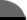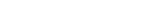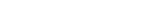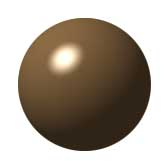Facts By Category:» Physics  » Astronomy  » Chemistry  » Biology  » Mathematics  » Geology  » Engineering  » Medicine  » Science  ScienceIQ Team:»Writers & EditorsxUmp.com
Science Supplies,

Physics & Astronomy
Online
How To Calculate The Circumference Of A CircleA circle is what you get if you take a straight line and bend it around so that its ends touch. You can demonstrate this by taking a piece of stiff wire and doing just that: bring the ends of the wire together end a circle shape will be formed. A true circle has a center, and every point on the line that got bent around to make the circle is exactly the same distance from this central point. That distance is called the radius of the circle. If you were to measure across the center of the circle from one edge to the other, you would be measuring the diameter of the circle. The diameter of a circle is exactly twice the length of the radius of the circle.

Because the length of the line that got bent around to make the circle is a very exact value and doesn't change, it will only make a circle of a certain size. The distance around the edge of the circle is called the circumference, and it can only be the same as the length of that line. There must therefore be a relation between the radius of a circle and the length of that line. The diameter of a circle is related to the circumference through a simple ratio: the value of 2p. If you were to take the original straight line and cut it into pieces so that each piece is as long as the radius of the circle, you would find in the end that you would have six equal pieces and one shorter piece. The circumference of a circle, then, is calculated by the general formula C = 2pr or C = pd.

As an example of how to use this formula, suppose you want to lay out a circular path in a garden to make a flower bed that will be 20 feet across. The path will be made of stones and you have enough stones to make 50 feet of the pathway. Will you need more stones, and if so, how much more will you need? (use p = 3.14) Substituting these values into the formula, you find that your path will be C = p X d. So C equals 3.14 X 20, or 62.8 feet long. Go get more stones.Richard M. J. Renneboog, MS Richard M. J. Renneboog is an independent private technical consultant and writer in both chemical and computer applications. Endeavors have included preparation of scripts for instructional and promotional video, corporate website design, curriculum development for training in advanced composites technology, and development.Bob Miller's Geometry for the Cluelessby Robert Miller
 Home | Privacy Policy | Cookie PolicyCopyright © 2002-2019 ScienceIQ.com - All Rights Reserved